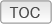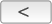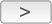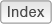Cakewalk // Documentation // Cakewalk // Backwards compatibilityTempo ► Backwards compatibility

When loading legacy Cakewalk and SONAR projects, the tempo map is automatically converted to a tempo envelope. The following Cakewalk.ini variables affect how tempo envelope shapes are preserved when opening Cakewalk 2021.04 (and later) projects in earlier versions.
 Variable Type Default value What it does Integer 1 (240 ticks / 16th note) Setting the value to 2 or 3 will increase the number of decimation points, making the tempo changes more accurate, but will slow down calculations when drawing envelopes. The values are as follows: 1 = 240 ticks (16th Note) 2 = 120 ticks (32nd Note) 3 = 60 ticks (64th Note) 4 = 30 ticks (128th Note) 5 = 15 ticks (256th Note) 6 = 12 ticks (320th Note) 7 = 6 ticks (640th Note) 8 = 3 ticks (1280th Note) Integer 1 (240 ticks / 16th note) This variable should be left at 1 in most cases in order to pick up most shapes. The values are as follows: 1 = 240 ticks (16th Note) 2 = 120 ticks (32nd Note) 3 = 60 ticks (64th Note) 4 = 30 ticks (128th Note) 5 = 15 ticks (256th Note) 6 = 12 ticks (320th Note) 7 = 6 ticks (640th Note) 8 = 3 ticks (1280th Note) TempoImportErrorThreshold=<0 - 250> Integer 10 This variable controls how close the tempo entries have to match points on a candidate curve before this curve is chosen. The best fit curve will always be chosen first, but this threshold determines whether to go with that curve or continue with discrete points. Valid entries are 0 to 250 (0.00 to 0.25). The default is 10, which equates to a difference of 0.01 of four point differences added together. A value of 0 effectively means import everything as discrete jump values.